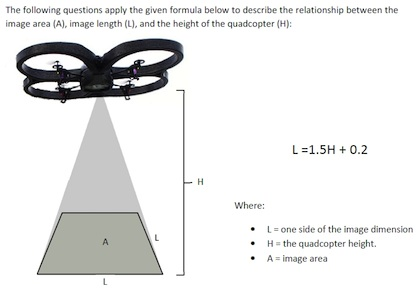## Friday, October 28, 2016

### Robots as Materials to Teach Math and Science

(from T.H.E Journal)... a interesting and series application of robots to teach important curriculum...

### Robot Set Helps Teach Math and Science

Can robots teach math? That's what RobotsLab is promoting with the release of its latest robotics product, the Box, a kit of hardware and curriculum intended to teach Algebra 1 and 2, physics, geometry, trigonometry, and pre-calculus for grades seven through 12. The Box comes in two versions, one for \$3,500, which includes four robots, a tablet computer that acts as the control station, accessories, and 50 lessons and quizzes. A "Deluxe" Box, which is \$3,999, includes access to additional lessons throughout the year and an extended warranty.

Each lesson is an app on the tablet that controls the robots in the package. Every module comes with an instructional video, a touch-based lesson, a quiz, and additional teaching materials. For example, a robotic ball is used in lessons on quadratic equations, statistics, and probability; a robotic arm helps teach geometry lessons on triangles and angles as well as trig lessons on arc, length, sine, and cosine; and a "quadcopter" provides physics lessons in gravity, acceleration, and frame of reference.RobotsLab's Box kits enable teachers to use robots to illustrate math concepts.
According to one lesson, the robots are used to "expose scientific principles providing a bridge between the abstract topics and the real world." In order to solve quizzes, the students have to work through equations, come to an understanding of physical forces, and perform scientific investigation.